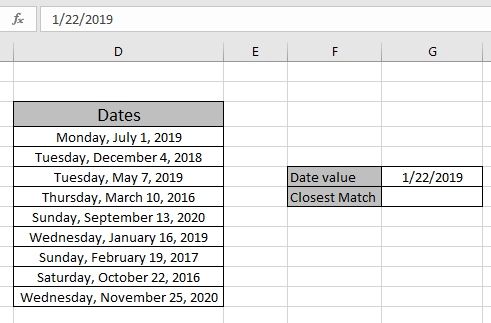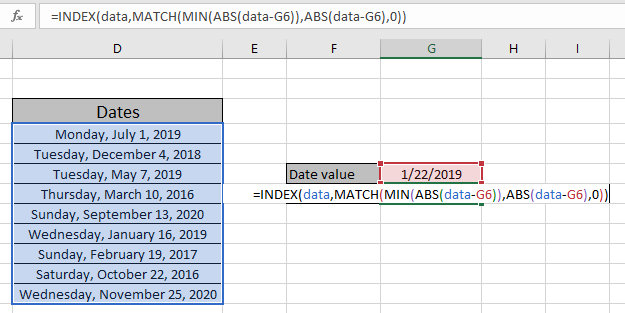# Lookup closest match using INDEX & MATCH function

In this article, we will learn how to Lookup closest match using INDEX & MATCH function in Excel.

Scenario:

For instance, We need to find the numerically closest match and its corresponding results from the table. We need some fixed formula which helps in finding the closest match as to get the required value.

How to Solve the Problem?

For the formula to understand first we need to revise a little about the following functions

Now we will make a formula using the above functions. MATCH function will return the index of the closest match from the range. The INDEX function takes the row index as argument and returns its corresponding required results.

Generic Formula:

{ = INDEX ( data , MATCH ( MIN ( ABS ( data - value ) ) , ABS ( data - value ) , 0 ) ) }

data : array of date values

value : given date value where result be closest to

match_type : 1 ( exact or next smallest ) or 0 ( exact match) or -1 ( exact or next largest )

DONOT use curly braces manually. Curly braces applied using the Ctrl + Shift + Enter in place of just Enter.

Example:

The above statements can be complicated to understand. So let’s understand this by using the formula in an example

Here we have some random date values and a given date value.Named range used for data (D4:D12).
Here we used the named range instead for cell reference array as it's easy to understand. We need to look for the date which is closest to the given date in G6 cell.

Use the above formula to get the closest date.
Use the formula :

{ = INDEX ( data , MATCH ( MIN ( ABS ( data - G6 ) ) , ABS ( data - G6 ) , 0 ) ) }

DONOT use curly braces manually. Curly braces applied using the Ctrl + Shift + Enter in place of just Enter.

Explanation:

• ABS function returns the positive integer after the subtraction performed over (data - G6) date values.
• ABS ( data - G6 ) makes an array by subtracting all date values from the given date value in G6 cell. The returned array is.

{ 160 ; 49 ; 105 ; 1048  ; 600 ; 6 ; 702 ; 822 ; 673 }

• MIN ( ABS ( data - G6 ) ) returns the closest value from the array returned from the above step.

= INDEX ( data , MATCH ( 6 , { 160 ; 49 ; 105 ; 1048  ; 600 ; 6 ; 702 ; 822 ; 673 } , 0 ) )

• MATCH function matches the closest minimum value match in the returned array and returns its row index to the INDEX function.
• The INDEX function finds the value having returned ROW index.data named range used for the dates array. Now use Ctrl + Shift + Enter in place for the just Enter top get the result as this is an array formula. Array formula is a formula where an array of values goes from one function result as argument to outer function.

As you can see from the above snapshot we obtained the closest matching from the given date in the table. Extract details from the table using the above stated formula.

Here are some observational noter while using the above formula.

Notes:

1. The function returns the #NA error if the lookup array argument to the MATCH function is not of same length of the table array.
2. The formula returns an error if lookup_value doesn't match the value in the table lookup_array.
3. The function matches exact value as the match type argument to the MATCH function is 0.
4. The lookup values can be given as cell reference or directly using quote symbol ( " ) in the formula as arguments.
5. DONOT use curly braces manually. Curly braces applied using the Ctrl + Shift + Enter in place of just Enter or else it returns error.

Hope you understood how to Lookup lowest or smallest match using the INDEX & MATCH function in excel. Explore more articles on Excel lookup value & Excel 2019 functions here. Please feel free to state your queries below in the comment box. We will certainly help you.

If you liked our blogs, share it with your friends on Facebook. And also you can follow us on Twitter and Facebook. We would love to hear from you, do let us know how we can improve, complement or innovate our work and make it better for you. Write us at info@exceltip.com

Related Articles

Use INDEX and MATCH to Lookup Value : INDEX & MATCH function to look up value as required.

SUM range with INDEX in Excel : Use INDEX function to find the SUM of the values as required.

How to use the INDEX function in Excel : Find the INDEX of array using the INDEX function explained with example.

How to use the MATCH function in Excel : Find the MATCH in the array using the INDEX value inside MATCH function explained with example.

How to use LOOKUP function in Excel : Find the lookup value in the array using the LOOKUP function explained with example.

How to use the VLOOKUP function in Excel : Find the lookup value in the array using the VLOOKUP function explained with example.

Popular Articles

50 Excel Shortcut to Increase Your Productivity

Edit a dropdown list

Absolute reference in Excel

If with conditional formatting

If with wildcards

The Excel 2019 CONCAT Function

The Excel 2019 MAXIFS Function

Terms and Conditions of use

The applications/code on this site are distributed as is and without warranties or liability. In no event shall the owner of the copyrights, or the authors of the applications/code be liable for any loss of profit, any problems or any damage resulting from the use or evaluation of the applications/code.